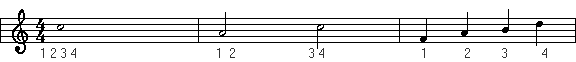Basic Counting:

One of the most obvious questions is how musicians know when to play. Well, its easy.. they learn to count the beats.

First let us present you with this.

1 whole note = 2 half notes = 4 quarter notes = 8 eighth notes = 16 sixteenth notes.
Keep that in mind while looking at these examples.First off, looking at the time signature you know that there are 4 quarter notes per measure.

In the first measure the whole note gets all the beats (1, 2, 3 and 4) because 1 whole note = 4 quarter notes, and there are a total of 4 quarter notes per measure.

In the second example, each half note gets 2 beats because 2 quarter notes = 1 half note.

In the third example, each quarter note gets its own beat because there are 4 quarter notes per measure (time sig).

Let's intermingle the 2 quarter notes and a half note.The half note get the first 2 beats, and each quarter its own beat. This makes sense because the 4/4 time signature means there is 4 quarter notes per measure. 2 quarter notes + 1 half note (which is really 2 quarters) = 4 quarter notes, the total number of quarter notes for that measure (time sig).

Lets add in the eighth notes.In this example there is something new. The + sign. It just means "and". If you said 1 + 2 + ... out loud it would sound like this.

1 and 2 and 3 and 4 and

Each eight note is 1/2 of a quarter note, therefore it takes 2 eighth notes to equal 1 quarter note.
Think of it like this: the 1 and the "and" are both half of one quarter note and together they form 1 quarter note and from the time sig we know there are 4 quarters per measure.

This may seem a little confusing now, but all of the sudden it will click. You will hit yourself in the head and wonder how you never understood it.

After you understand this, go on to the counting basics 2 page. ;-)

 Back to the... On to... Types of Rests Basic Counting 2

[ Introduction | Clefs | Time Signatures | Types of Notes | Types of Rests | Basic Counting I ]
[ Basic Counting II | Symbols | Shaping | Return to Education Section ]

Learning to Read Music, Created by Kevin Lux for